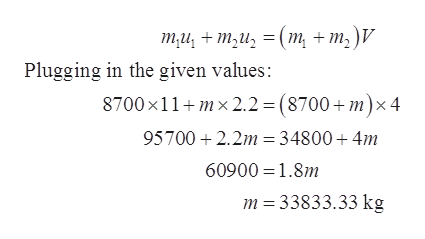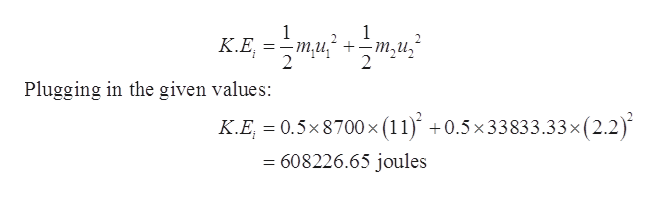# On the planet Gizmo, the inhabitants travel by high speed trains that run on air tracks much like the air track you used in lab. A train car with a mass of 8700 kg is traveling at 11.0 m/s when it strikes a second car moving in the same direction at 2.2 m/s. The two stick together and move off with a speed of 4.00 m/s. What is the mass of the second car? Explain your reasoning. How much kinetic energy was lost in the collision?

Question

On the planet Gizmo, the inhabitants travel by high speed trains that run on air tracks much like the air track you used in lab. A train car with a mass of 8700 kg is traveling at 11.0 m/s when it strikes a second car moving in the same direction at 2.2 m/s. The two stick together and move off with a speed of 4.00 m/s. What is the mass of the second car? Explain your reasoning. How much kinetic energy was lost in the collision?

check_circle

Step 1

Given values:

Mass of the first train car, m1 = 8700 kg

Speed of the first train car, u1 = 11.0 m/s

Let the mass of the second train car, m2 = m

Speed of the first train car, u2 = 2.2 m/s

Common speed of the both car V = 4.00 m/s

Step 2

By the conservation of momentum:

Total initial momentum = Total final momentumhelp_outlineImage Transcriptioncloseти, + т,и, 3 (т, + т,)V Plugging in the given values: 8700 x11 mx 2.2 = (8700+ m)x4 95700 2.2m 34800 4m 60900 1.8m m 33833.33 kg fullscreen
Step 3

Kinetic energy lost:

Initial...help_outlineImage Transcriptionclose2 -ти,* + -т,и, = Plugging in the given values: K.E 0.5x8700x (11) +0.5x33833.33x(2.2) - 608226.65 joules fullscreen

### Want to see the full answer?

See Solution

#### Want to see this answer and more?

Solutions are written by subject experts who are available 24/7. Questions are typically answered within 1 hour.*

See Solution
*Response times may vary by subject and question.
Tagged in

### Other